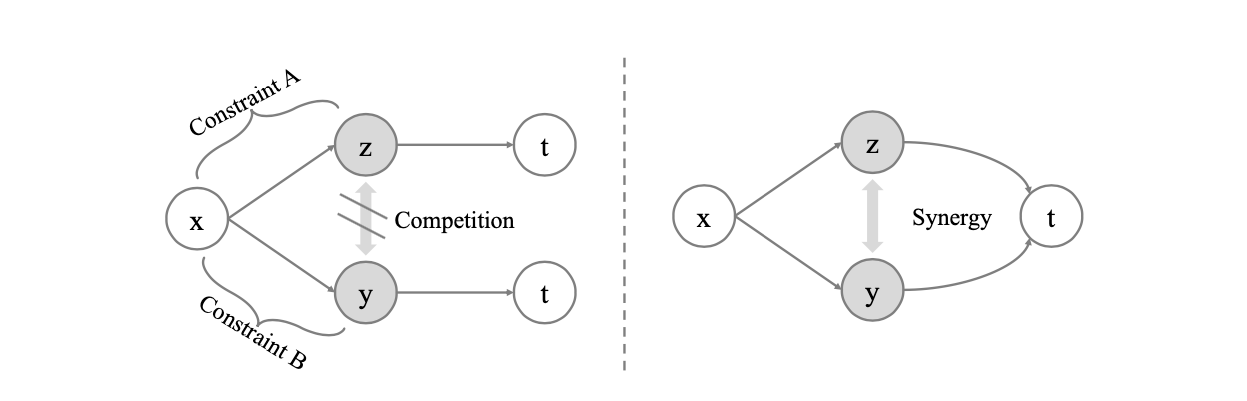N1H111SM's Miniverse

# Information Competing Process for Learning Diversified Representations

2020/05/27 ShareMaterials

# Motivation

Aiming to enrich the information carried by feature representations, ICP separates a representation into two parts with different mutual information constraints. The separated parts are forced to accomplish the downstream task independently in a competitive environment which prevents the two parts from learning what each other learned for the downstream task. Such competing parts are then combined synergistically to complete the task.

## Representation Collaboration

The Competitive Collaboration method is the most relevant to our work. It defines a three-player game with two competitors and a moderator, where the moderator takes the role of a critic and the two competitors collaborate to train the moderator. Unlike Competitive Collaboration, the proposed ICP enforces two (or more) representation parts to be complementary through different mutual information constraints for the same downstream task by a competitive environment, which endows the capability of learning more discriminative and disentangled representations.

# Methods

## Seperating Representations

Directly separate the representation $r$ into two parts $[z, y]$. Specifically, we constrain the information capacity of representation part $z$ while increasing the information capacity of representation part $y$.

## Representation Competition

ICP prevents $z$ and $y$ from knowing what each other learned for the downstream task, which is realized by enforcing $z$ and $y$ independent of each other.

where $\alpha^\prime > 1$ and $\beta^\prime < 1$. 如果把$z$和$y$分别看作数据的两个view，那么中间两项就代表不同view和$X$ 之间的相关度；第一项代表全局representation和input之间的相关度；而最后一项就是view之间的InfoMin项。## Minimizing MI

Let $Q(z)$ be a variational approximation of $P(z)$, we have:

which enforces the extracted $z$ conditioned on $x$ to a predefined distribution $Q(z)$ such as a standard Gaussian distribution.